# RS Aggarwal Solutions for Class 9 Chapter 5: Coordinate Geometry

The position of points in a plane can be found with the help of Coordinate Geometry with the set of numbers called Coordinates. Coordinate Geometry in modern days is also famous as Cartesian or Analytic Geometry. The plane in which all the points are located in coordinate geometry is known as coordinate plane.

RS Aggarwal Solutions for Class 9 helps students to have a good grasp and better understanding of all the concepts in the textbook. RS Aggarwal Solutions for Class 9 Chapter 5 Coordinate Geometry are provided here.

## RS Aggarwal Solutions for Class 9 Chapter 5 : Coordinate Geometry Download PDF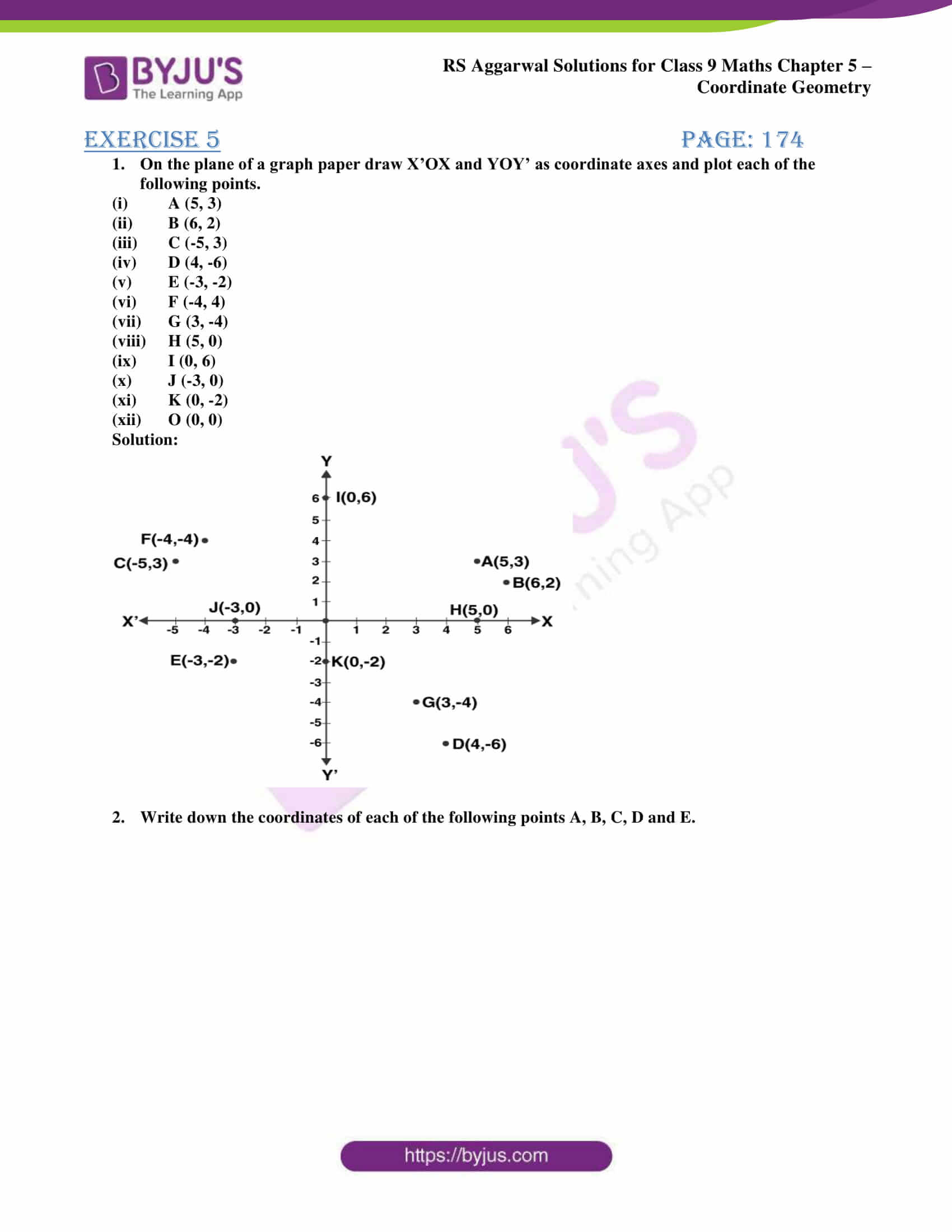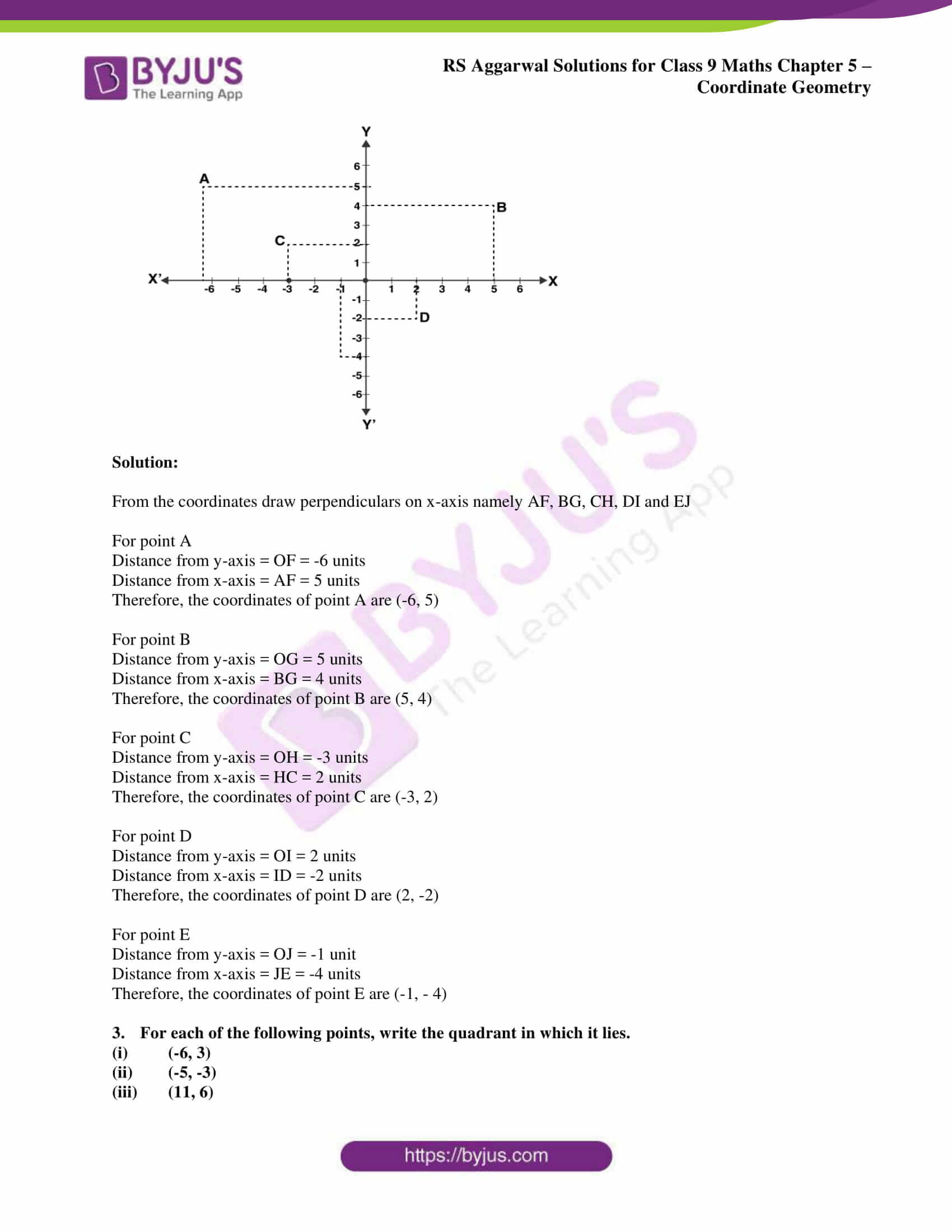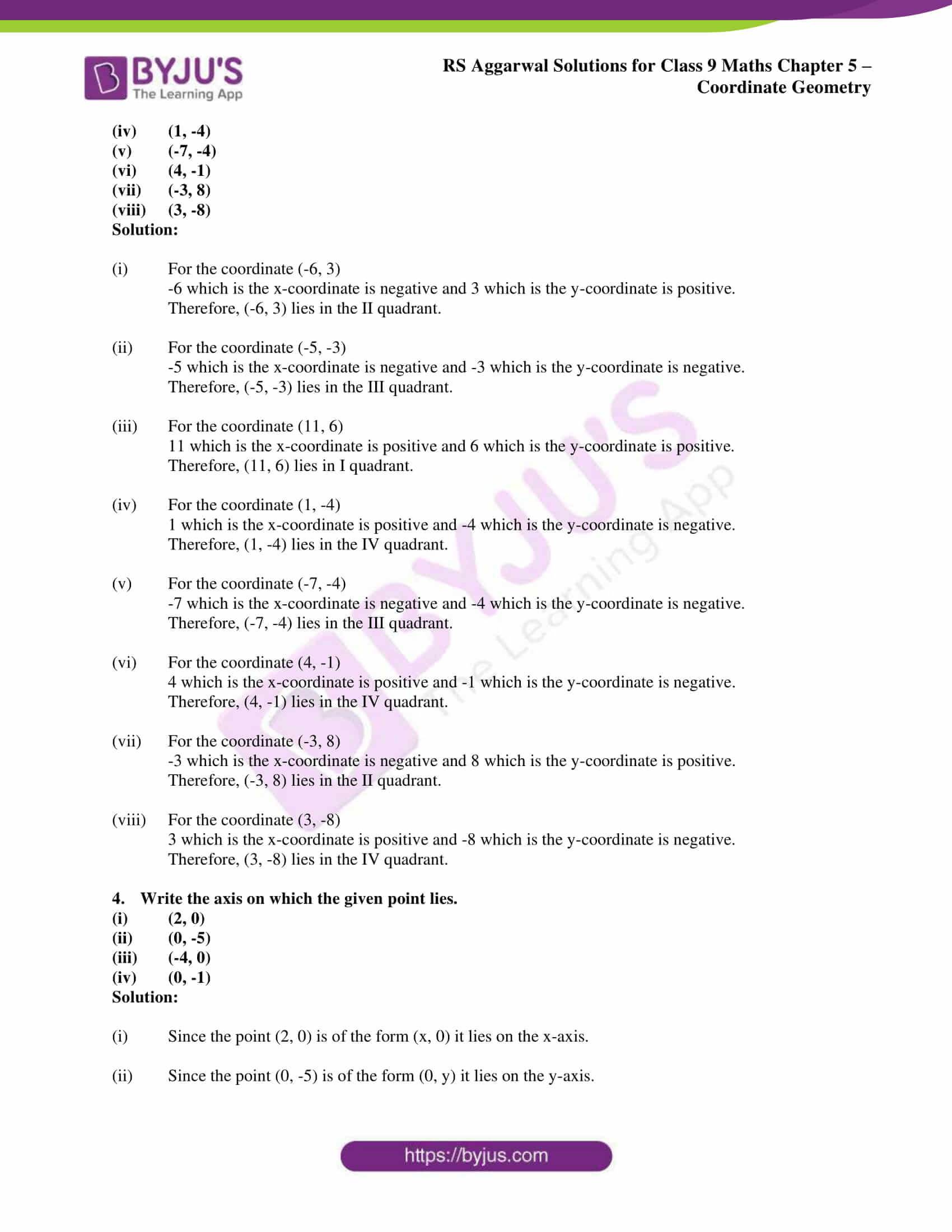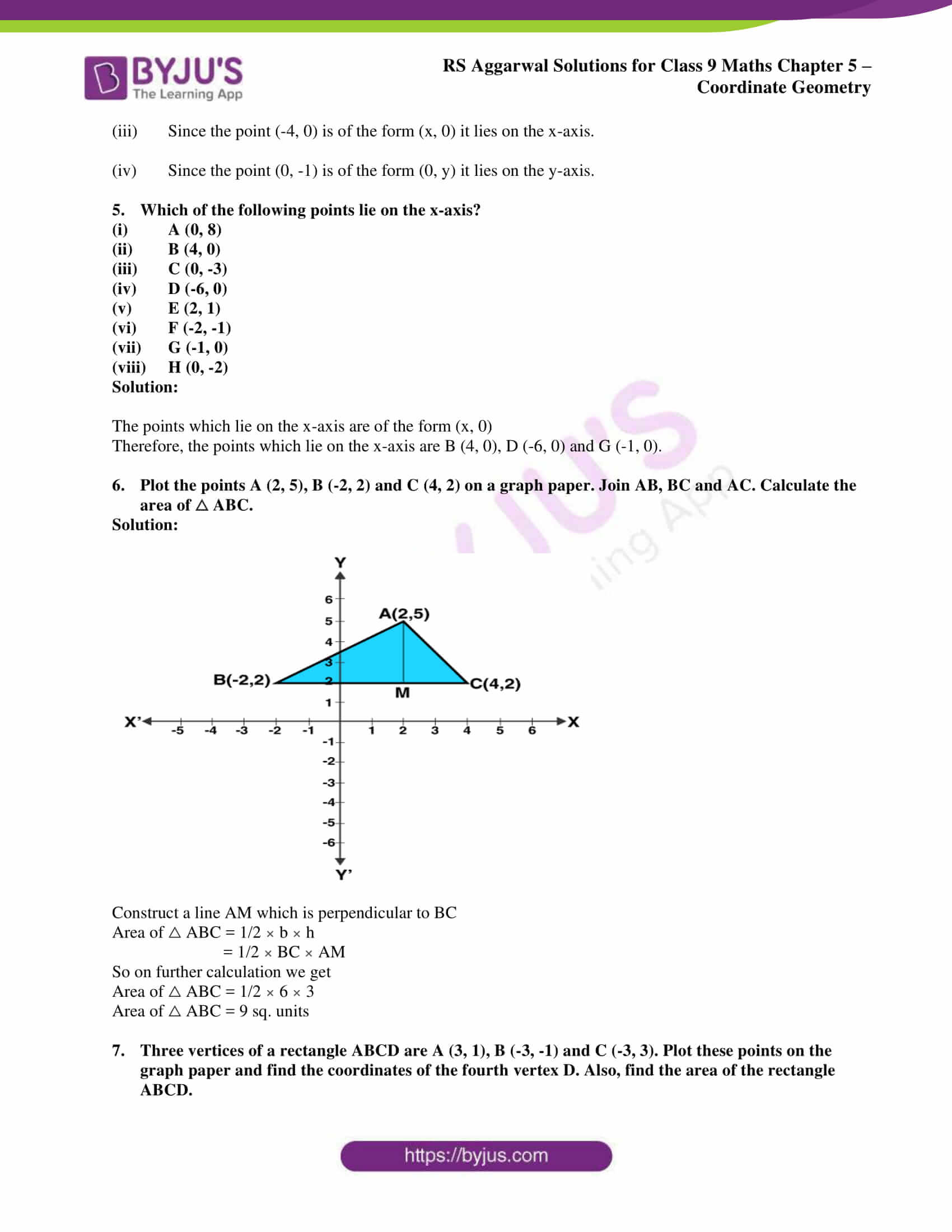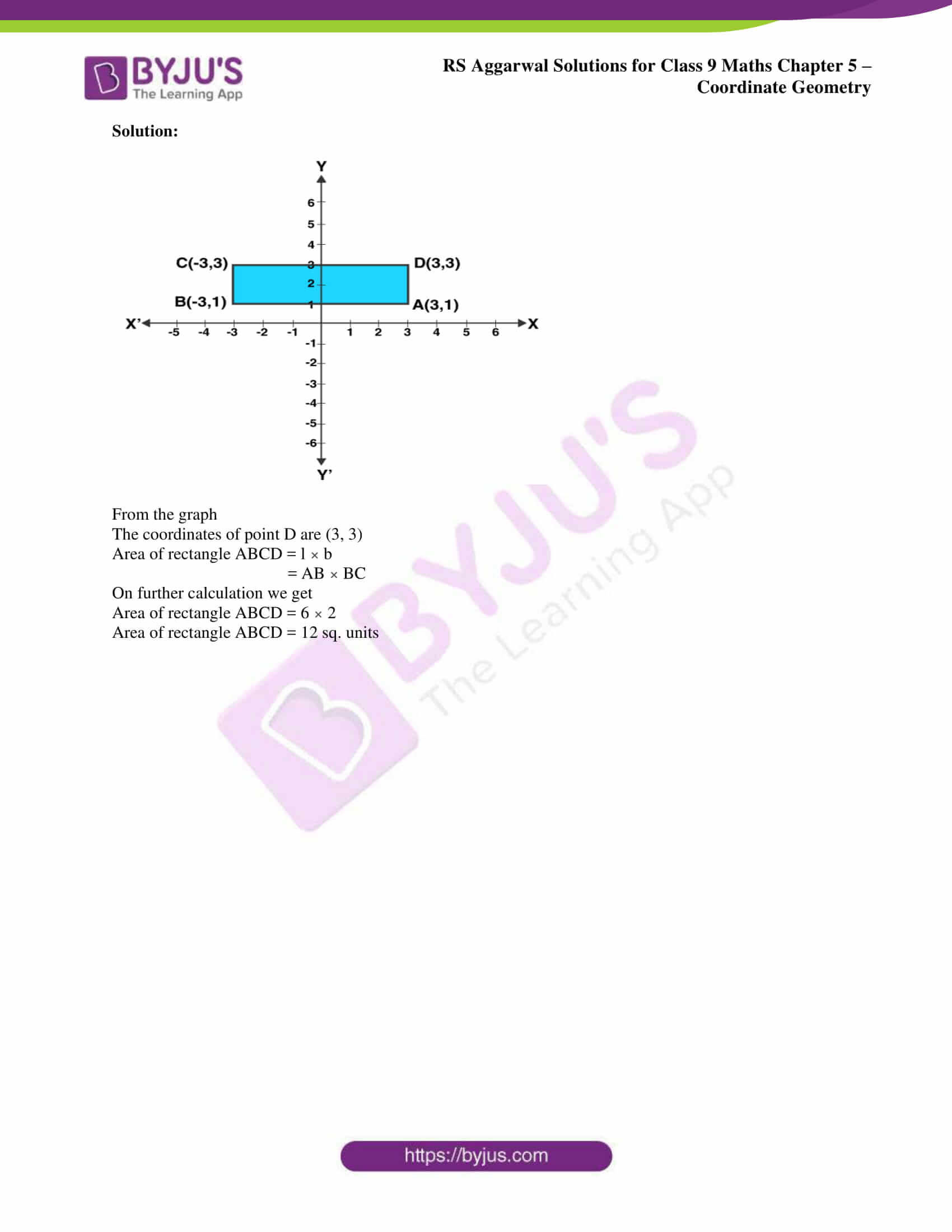## Access RS Aggarwal Solutions for Class 9 Chapter 5: Coordinate Geometry

Exercise 5 PAGE: 174

1. On the plane of a graph paper draw X’OX and YOY’ as coordinate axes and plot each of the following points.

1. A (5, 3)
2. B (6, 2)
3. C (-5, 3)
4. D (4, -6)
5. E (-3, -2)
6. F (-4, 4)
7. G (3, -4)
8. H (5, 0)
9. I (0, 6)
10. J (-3, 0)
11. K (0, -2)
12. O (0, 0)

Solution: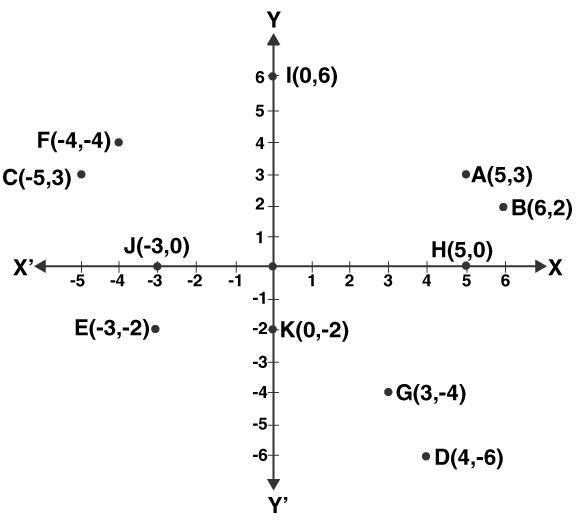2. Write down the coordinates of each of the following points A, B, C, D and E.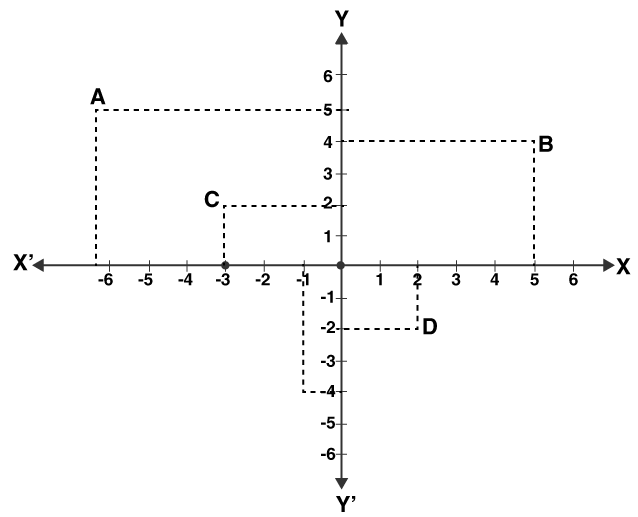Solution:

From the coordinates draw perpendiculars on x-axis namely AF, BG, CH, DI and EJ

For point A

Distance from y-axis = OF = -6 units

Distance from x-axis = AF = 5 units

Therefore, the coordinates of point A are (-6, 5)

For point B

Distance from y-axis = OG = 5 units

Distance from x-axis = BG = 4 units

Therefore, the coordinates of point B are (5, 4)

For point C

Distance from y-axis = OH = -3 units

Distance from x-axis = HC = 2 units

Therefore, the coordinates of point C are (-3, 2)

For point D

Distance from y-axis = OI = 2 units

Distance from x-axis = ID = -2 units

Therefore, the coordinates of point D are (2, -2)

For point E

Distance from y-axis = OJ = -1 unit

Distance from x-axis = JE = -4 units

Therefore, the coordinates of point E are (-1, – 4)

3. For each of the following points, write the quadrant in which it lies.

1. (-6, 3)
2. (-5, -3)
3. (11, 6)
4. (1, -4)
5. (-7, -4)
6. (4, -1)
7. (-3, 8)
8. (3, -8)

Solution:

1. For the coordinate (-6, 3)

-6 which is the x-coordinate is negative and 3 which is the y-coordinate is positive.

Therefore, (-6, 3) lies in the II quadrant.

2. For the coordinate (-5, -3)

-5 which is the x-coordinate is negative and -3 which is the y-coordinate is negative.

Therefore, (-5, -3) lies in the III quadrant.

3. For the coordinate (11, 6)

11 which is the x-coordinate is positive and 6 which is the y-coordinate is positive.

Therefore, (11, 6) lies in I quadrant.

4. For the coordinate (1, -4)

1 which is the x-coordinate is positive and -4 which is the y-coordinate is negative.

Therefore, (1, -4) lies in the IV quadrant.

5. For the coordinate (-7, -4)

-7 which is the x-coordinate is negative and -4 which is the y-coordinate is negative.

Therefore, (-7, -4) lies in the III quadrant.

6. For the coordinate (4, -1)

4 which is the x-coordinate is positive and -1 which is the y-coordinate is negative.

Therefore, (4, -1) lies in the IV quadrant.

7. For the coordinate (-3, 8)

-3 which is the x-coordinate is negative and 8 which is the y-coordinate is positive.

Therefore, (-3, 8) lies in the II quadrant.

8. For the coordinate (3, -8)

3 which is the x-coordinate is positive and -8 which is the y-coordinate is negative.

Therefore, (3, -8) lies in the IV quadrant.

4. Write the axis on which the given point lies.

1. (2, 0)
2. (0, -5)
3. (-4, 0)
4. (0, -1)

Solution:

1. Since the point (2, 0) is of the form (x, 0) it lies on the x-axis.
2. Since the point (0, -5) is of the form (0, y) it lies on the y-axis.
3. Since the point (-4, 0) is of the form (x, 0) it lies on the x-axis.
4. Since the point (0, -1) is of the form (0, y) it lies on the y-axis.

5. Which of the following points lie on the x-axis?

1. A (0, 8)
2. B (4, 0)
3. C (0, -3)
4. D (-6, 0)
5. E (2, 1)
6. F (-2, -1)
7. G (-1, 0)
8. H (0, -2)

Solution:

The points which lie on the x-axis are of the form (x, 0)

Therefore, the points which lie on the x-axis are B (4, 0), D (-6, 0) and G (-1, 0).

6. Plot the points A (2, 5), B (-2, 2) and C (4, 2) on a graph paper. Join AB, BC and AC. Calculate the area of △ ABC.

Solution: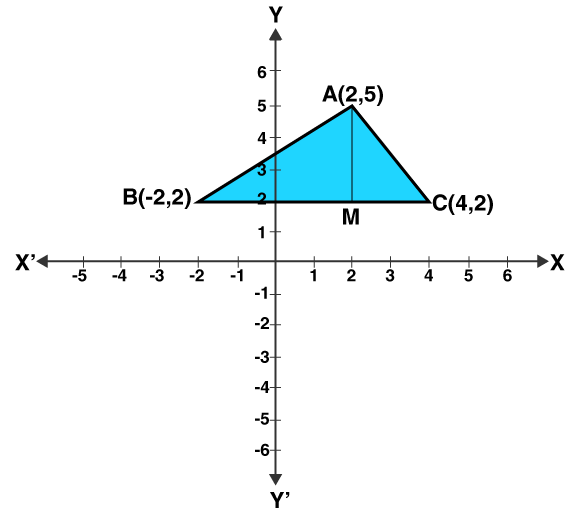Construct a line AM which is perpendicular to BC

Area of △ ABC = 1/2 × b × h

= 1/2 × BC × AM

So on further calculation we get

Area of △ ABC = 1/2 × 6 × 3

Area of △ ABC = 9 sq. units

7. Three vertices of a rectangle ABCD are A (3, 1), B (-3, -1) and C (-3, 3). Plot these points on the graph paper and find the coordinates of the fourth vertex D. Also, find the area of the rectangle ABCD.

Solution: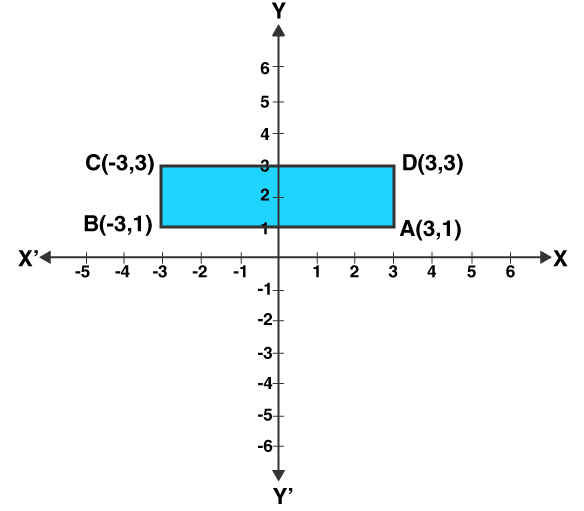From the graph

The coordinates of point D are (3, 3)

Area of rectangle ABCD = l × b

= AB × BC

On further calculation we get

Area of rectangle ABCD = 6 × 2

Area of rectangle ABCD = 12 sq. units

### RS Aggarwal Solutions for Class 9 Maths Chapter 5: Coordinate Geometry

Chapter 5, Coordinate Geometry, has 1 exercise which covers some of the relevant topics of major importance in RS Aggarwal Solutions for Class 9. Some of the topics which are discussed in this chapter are as listed below:

• Introduction
• Cartesian Coordinates
• Coordinate Geometry
• Coordinate System
• Coordinates of a point in a plane
• Points on Coordinate Axes

### RS Aggarwal Solutions Class 9 Maths Chapter 5 – Coordinate Geometry

In order to help students perform well in the exams we have provided them with complete solutions for class 9 Maths Chapter 5 Coordinate Geometry. The answers are solved in various methods by our subject experts to make it easier for the students to improve their performance in exams.

Coordinate Geometry has applications in major fields like Aviation, Differential Geometry, Rocketry, Computational Geometry, Space Flight and Space Science. Students can download RS Aggarwal solutions for Class 9 PDF in this page as a reference for better understanding of concepts covered in this chapter.